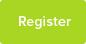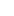# Tutor Hunt Questions

A particleis travelling is a straightline with acceleration a passes A whilst movingwith velocityu , when it reaches a point B it has velocityv and at this point its acceleration changes to -a show that when it again passes by A its
Speed is squre root of 2v^2-u^2
2 years ago

Physics Question asked by Nour Elhuda

Please enter your response to the question below. The student will get a notification as soon your response has been approved by our moderation team.

First part path AB: using suvat eqns get v^2= u^2 + 2as where s is the fixed displacement AB (remember all these quantities are vectors so +ve is direction from AB).

so solving for s= (v^2 - u^2)/2a

[each part of motion has its own set of suvat values]
Second part, particle must travel from pt B back again to A (eventually) so its displacement here will be -s (note the negative value)

using v`^2= u^2 + 2as again, but this time substituting v for u, -a for a and -s for s, then letting v` be the final velocity as it returns to pt.A:

v`^2= v^2 +2(-a)(-s) = v^2 +2as = v^2 + 2a[(v^2 - u^2)/2a]
= v^2 + [v^2 - u^2] = 2v^2 - u^2

taking sqr. get speed |v`|...

speed = SQR[2v^2 - u^2] as rqd.

note that the displacement s has same magnitude both parts of the problem, we are using displacement not distance.
Answered by Tom | 2 years ago
This is a SUVAT problem.

V^2 = U^2 + 2as , where s = dsitance A to B.
Now on the way back, B to A, its initial speed U = V.

i.e. After B, The paticle will slow to zero, stop and return to point B with
speed V now in the direction towards A by symmetry.

Lets call its new final velocity when it returns to A, V1.

V1^2 = V^2 + 2as ,

But  ==> 2as = V^2-U^2,

Sub this in ,

V1^2 = V^2 + (V^2-U^2),

V1^2 = 2V^2 -U^2 QED